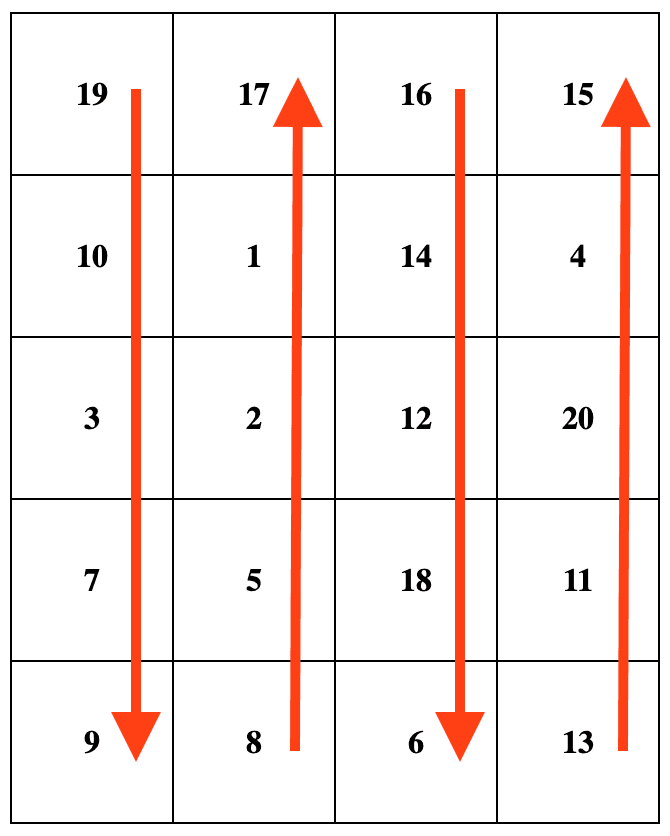# 2624. Snail Traversal

## Description

Write code that enhances all arrays such that you can call the snail(rowsCount, colsCount) method that transforms the 1D array into a 2D array organised in the pattern known as snail traversal order. Invalid input values should output an empty array. If rowsCount * colsCount !== nums.length, the input is considered invalid.

Snail traversal order starts at the top left cell with the first value of the current array. It then moves through the entire first column from top to bottom, followed by moving to the next column on the right and traversing it from bottom to top. This pattern continues, alternating the direction of traversal with each column, until the entire current array is covered. For example, when given the input array [19, 10, 3, 7, 9, 8, 5, 2, 1, 17, 16, 14, 12, 18, 6, 13, 11, 20, 4, 15] with rowsCount = 5 and colsCount = 4, the desired output matrix is shown below. Note that iterating the matrix following the arrows corresponds to the order of numbers in the original array.Example 1:

Input:
nums = [19, 10, 3, 7, 9, 8, 5, 2, 1, 17, 16, 14, 12, 18, 6, 13, 11, 20, 4, 15]
rowsCount = 5
colsCount = 4
Output:
[
[19,17,16,15],
[10,1,14,4],
[3,2,12,20],
[7,5,18,11],
[9,8,6,13]
]


Example 2:

Input:
nums = [1,2,3,4]
rowsCount = 1
colsCount = 4
Output: [[1, 2, 3, 4]]


Example 3:

Input:
nums = [1,3]
rowsCount = 2
colsCount = 2
Output: []
Explanation: 2 multiplied by 2 is 4, and the original array [1,3] has a length of 2; therefore, the input is invalid.


Constraints:

• 0 <= nums.length <= 250
• 1 <= nums[i] <= 1000
• 1 <= rowsCount <= 250
• 1 <= colsCount <= 250

## Solutions

• declare global {
interface Array<T> {
snail(rowsCount: number, colsCount: number): number[][];
}
}

Array.prototype.snail = function (
rowsCount: number,
colsCount: number,
): number[][] {
if (rowsCount * colsCount !== this.length) {
return [];
}
const ans: number[][] = Array.from({ length: rowsCount }, () =>
Array(colsCount),
);
for (let h = 0, i = 0, j = 0, k = 1; h < this.length; ++h) {
ans[i][j] = this[h];
i += k;
if (i === rowsCount || i === -1) {
i -= k;
k = -k;
++j;
}
}
return ans;
};

/**
* const arr = [1,2,3,4];
* arr.snail(1,4); // [[1,2,3,4]]
*/